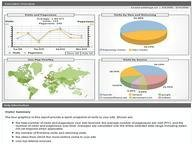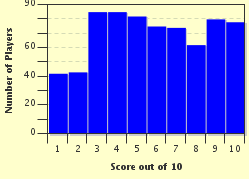FREE! Click here to Join FunTrivia. Thousands of games, quizzes, and lots more!# Pick A Card, Any Card! Trivia Quiz

### These will be basic probability questions based on picking cards from a standard 52 card deck. Be careful, some questions involve conditional probability! Good luck!

A multiple-choice quiz by rodney_indy. Estimated time: 5 mins.

Author
rodney_indy
Time
5 mins
Type
Multiple Choice
Quiz #
269,306
Updated
Dec 03 21
# Qns
10
Difficulty
Tough
Avg Score
5 / 10
Plays
3169
Awards
Top 20% Quiz
Last 3 plays: Guest 84 (9/10), Guest 111 (0/10), Guest 120 (4/10).
This quiz has 2 formats: you can play it as a or as shown below.
Scroll down to the bottom for the answer key.
1. A card is selected at random from a standard 52 card deck. Assuming all cards are equally likely to be selected, what is the probability that a red king was selected? Hint

1/52
15/26
1/13
1/26

#### NEXT>

2. A card is selected at random from a standard 52 card deck. Assuming all cards are equally likely to be selected, what is the probability that a red card or a king was selected? Hint

1/2
15/26
7/13
17/26

#### NEXT>

3. A card is selected at random from a standard 52 card deck. Assuming all cards are equally likely to be selected, what is the probability that a red card was selected given that a king was selected? Hint

1/26
1/13
1/2
1/4

#### NEXT>

4. A card is selected at random from a standard 52 card deck. Assuming all cards are equally likely to be selected, what is the probability that a king was selected given that a red card was selected? Hint

1/13
1/2
1/26
1/4

#### NEXT>

5. A card is selected at random from a standard 52 card deck. We are told that a king was not selected. Assuming all cards are equally likely to be selected, what is the probability that the queen of hearts was selected? Hint

1/48
1/52
1/13
1/39

#### NEXT>

6. Suppose two cards are drawn from a standard 52 card deck without replacement (which means when we draw a card, we don't put it back). Assuming all cards are equally likely to be selected, what is the probability that the second card is an ace given that the first card is an ace? Hint

1/17
3/52
1/221
1/13

#### NEXT>

7. Suppose two cards are drawn from a standard 52 card deck without replacement (which means when we draw a card, we don't put it back). Assuming all cards are equally likely to be selected, what is the probability that the second card is not an ace given that the first card is not an ace? Hint

47/51
1/4
1/13
16/17

#### NEXT>

8. Suppose two cards are drawn from a standard 52 card deck without replacement (which means when we draw a card, we don't put it back). Assuming all cards are equally likely to be selected, what is the probability that both cards are red? Hint

1/4
25/102
25/2704
13/50

#### NEXT>

9. Suppose two cards are drawn from a standard 52 card deck without replacement (which means when we draw a card, we don't put it back). Assuming all cards are equally likely to be selected, what is the probability that both cards are not aces? Hint

188/221
15/16
144/169
12/13

#### NEXT>

10. Suppose two cards are drawn from a standard 52 card deck without replacement (which means when we draw a card, we don't put it back). Assuming all cards are equally likely to be selected, what is the probability that the second card is an ace given that the first card is a club? Hint

3/51
1/13
4/51
2/25

 (Optional) Create a Free FunTrivia ID to save the points you are about to earn:Select a User ID:Choose a Password:Your Email:

Most Recent Scores
Sep 28 2023 : Guest 84: 9/10
Sep 27 2023 : Guest 111: 0/10
Sep 19 2023 : Guest 120: 4/10
Sep 07 2023 : Guest 199: 5/10
Aug 20 2023 : Guest 110: 8/10
Aug 19 2023 : Guest 130: 1/10
Aug 17 2023 : Guest 72: 1/10
Aug 14 2023 : Guest 120: 0/10
Aug 06 2023 : Guest 86: 10/10

Score DistributionQuiz Answer Key and Fun Facts
1. A card is selected at random from a standard 52 card deck. Assuming all cards are equally likely to be selected, what is the probability that a red king was selected?

There are four kings in the deck - 2 are red and 2 are black. So the probability that a red king was selected is 2/52 = 1/26.
2. A card is selected at random from a standard 52 card deck. Assuming all cards are equally likely to be selected, what is the probability that a red card or a king was selected?

Of the 52 cards in the deck, 26 are red, 4 are kings, and 2 are red kings. Hence the number of cards that are red or are a king are 26 + 4 - 2 = 28. We subtract 2 since the 2 red kings are being counted twice. So the probability that a red card or a king was selected is 28/52 = 7/13.
3. A card is selected at random from a standard 52 card deck. Assuming all cards are equally likely to be selected, what is the probability that a red card was selected given that a king was selected?

This is a conditional probability question. We are given that a king was selected. There are 4 kings in the deck. Of the 4 kings, 2 of them are red. So the probability that a red card was selected given that a king was selected is 2/4 = 1/2.
4. A card is selected at random from a standard 52 card deck. Assuming all cards are equally likely to be selected, what is the probability that a king was selected given that a red card was selected?

This is a conditional probability question. We are given that a red card was selected. There are 26 red cards in the deck. Of the 26 red cards, 2 of them are kings. So the probability that a king was selected given that a red card was selected is 2/26 = 1/13.
5. A card is selected at random from a standard 52 card deck. We are told that a king was not selected. Assuming all cards are equally likely to be selected, what is the probability that the queen of hearts was selected?

This is a conditional probability question. We are given that a king was not selected. There are 48 cards in the deck that are not kings. Of the 48 cards that aren't kings, 1 of them is the queen of hearts. So the probability that the queen of hearts was selected given that a king was not selected is 1/48.
6. Suppose two cards are drawn from a standard 52 card deck without replacement (which means when we draw a card, we don't put it back). Assuming all cards are equally likely to be selected, what is the probability that the second card is an ace given that the first card is an ace?

This is a conditional probability question. We are given that the first card is an ace. There are 51 possibilities for the second card, 3 of which are aces (we are given the first card was an ace). So the probability that the second card is an ace given that the first card is an ace is 3/51 = 1/17.
7. Suppose two cards are drawn from a standard 52 card deck without replacement (which means when we draw a card, we don't put it back). Assuming all cards are equally likely to be selected, what is the probability that the second card is not an ace given that the first card is not an ace?

This is a conditional probability question. We are given that the first card is not an ace. There are 4 aces in the deck, so there are 48 cards that are not aces. After the first card is drawn, there are 51 cards left in the deck. Among those 51 cards, 47 are not aces. Hence the probability is 47/51.
8. Suppose two cards are drawn from a standard 52 card deck without replacement (which means when we draw a card, we don't put it back). Assuming all cards are equally likely to be selected, what is the probability that both cards are red?

There are 26 red cards in the deck. So the probability that the first card is red is 26/52 = 1/2. The probability that the second card is red given that the first card is red is 25/51, since there are 25 red cards left out of the remaining 51 cards. So the probability that both cards are red is (1/2) * (25/51) = 25/102.

Another solution: The sample space consists of C(52,2) = 52*51/2 = 26*51 different two card hands. The number of ways we can select 2 red cards from 26 red cards is C(26,2) = 26*25/2 = 13*25. So the probability is (13*25)/(26*51) = 25/102.
9. Suppose two cards are drawn from a standard 52 card deck without replacement (which means when we draw a card, we don't put it back). Assuming all cards are equally likely to be selected, what is the probability that both cards are not aces?

There are 48 cards in the deck that are not aces. The probability that the first card selected is not an ace is 48/52 = 12/13. Since the first card was not an ace, there are 47 cards that are not aces among the remaining 51 cards in the deck. So the probability that the second card is not an ace given that the first card is not an ace is 47/51. Therefore, the probability that both cards are not aces is (12/13)*(47/51) = 188/221.

Another solution: The sample space consists of C(52,2) = 52*51/2 = 26*51 different two card hands. There are 48 cards in the deck that are not aces, we can select two cards that are not aces from the 48 that are not aces in C(48,2) = 48*47/2 = 24*47 different ways. Hence the probability is (24*47)/(26*51) = (4*47)/(13*17) = 188/221.
10. Suppose two cards are drawn from a standard 52 card deck without replacement (which means when we draw a card, we don't put it back). Assuming all cards are equally likely to be selected, what is the probability that the second card is an ace given that the first card is a club?

Let E be the event "the first card is a club." Let F be the event "the second card is an ace." By the formula for conditional probability,

Pr(F|E) = Pr(F intersect E)/Pr(E).

The probability in the denominator is the easy one: 13 of the 52 cards in the deck are clubs, so Pr(E) = 13/52. We need to compute Pr(F intersect E). Note that there are two ways the first card could be a club and the second card could be an ace:

Case 1: The first card is the ace of clubs and the second card is not the ace of clubs, which has probability (1/52)*(3/51)=3/(52*51).
Case 2: The first card is a club other than the ace of clubs and the second card is an ace, which has probability (12/52)*(4/51)=48/(52*51).

This means the probability of F intersect E is just the sum of these probabilities, which is 51/(52*51) = 1/52. So by the formula for conditional probability, Pr(F|E)=Pr(F intersect E)/Pr(E)=(1/52)/(13/52)=1/13.

I hope you enjoyed this quiz! Thanks for playing!
Source: Author rodney_indy

This quiz was reviewed by FunTrivia editor crisw before going online.
Any errors found in FunTrivia content are routinely corrected through our feedback system.